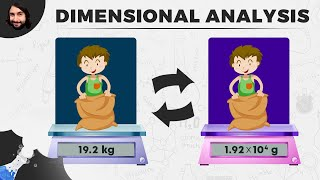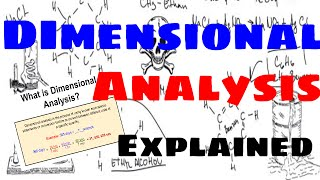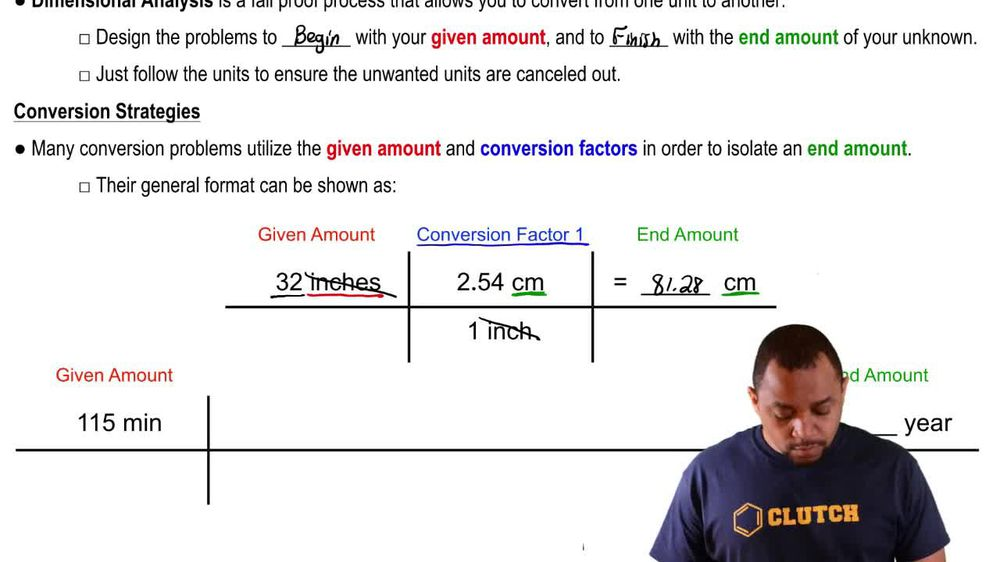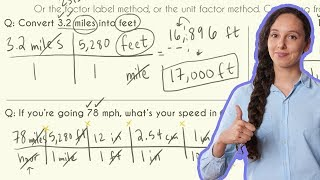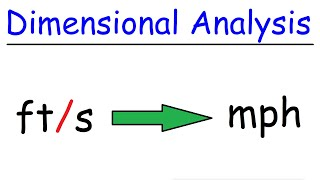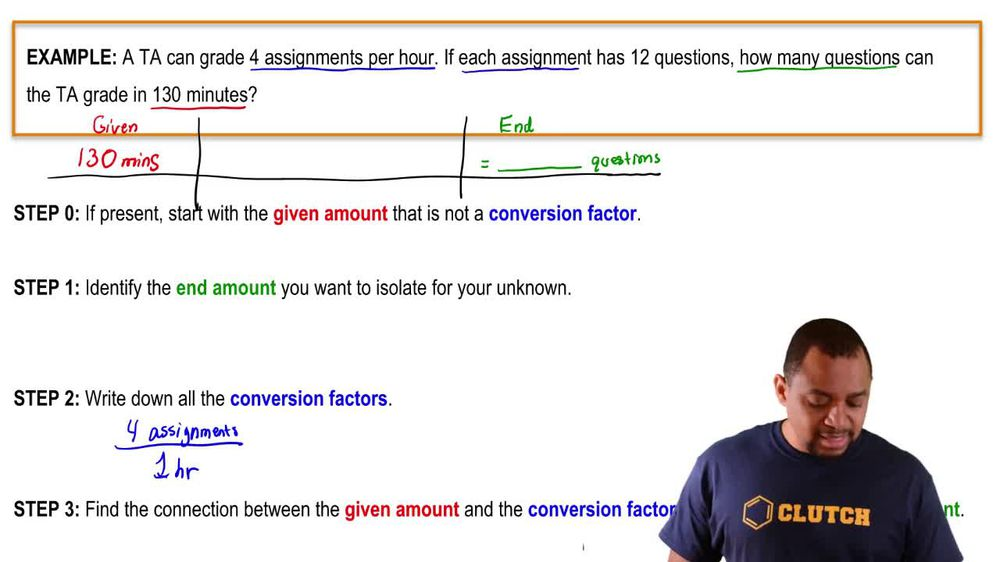Start typing, then use the up and down arrows to select an option from the list.
1. 1. Intro to General Chemistry2. Dimensional Analysis
Problem

# The estimated concentration of gold in the oceans is 1.0 * 10^-11 g/mL. (b) Assuming that the volume of the oceans is 1.3 * 10^21 L, estimate the amount of dissolved gold in grams in the oceans.

Relevant Solution1m
Play a video:
Hey everyone, we're told that the concentration of dissolved iron in the pacific ocean is two point oh times 10 to the negative nine g per mil leader. If the volume of the pacific ocean is estimated to be 7.14 times 10 to liters, calculate the mass in grams of dissolved iron to calculate our mass of dissolved iron. We can go ahead and start off with our 7.14 times 10 to the 20 leaders. Using our dimensional analysis, we want to convert this into milliliters. We know that we have 10 to the third milliliters per one liter. Now, using the concentration of dissolved iron, we know that we have two point oh times 10 to the negative nine g per one millimeter. So when we calculate this out and cancel out all of our units, we end up with a mass of 1.4 times 10 to the 15 g. And this is going to be our final answer. Now, I hope that made sense. And let us know if you have any questions